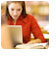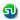### Permutation Combination questions, practice tests, sample problems, question bank : Ascent - MBA TANCET, XAT Classes

 Home TANCET Classes GMAT Coaching Online GMAT Course CAT Classes TANCET Study Material
 TANCET '18 Classroom ProgramWeekend and weekday classes for TANCET MBA @ Chennai. At Nungambakkam and Velachery.Other Courses TANCET Correspondence Course CAT Classes & Courses GMAT Classes Chennai Online GMAT Course GRE Classes Chennai CBSE Math Online Tuition SAT Classes Other Links Ascent TANCET Toppers Testimonials CAT, TANCET Questions Careers @ Ascent Contact Us +91 44 4500 8484 +91 96000 48484 ascent@ascenteducation.com Postal Address Facebook / Twitter / Blog / Videos
You are here: Home  »  XAT, TANCET, Test Prep Questions Answers »  Permutation Combination

# Permutation Combination - Practice Questions

A collection of questions that typically appear from the topic of Permutation and Combination.
1. Question 1: A college has 10 basketball players. A 5-member team and a captain will be selected out of these 10 players. How many different selections can be made?

2. Question 2:  Badri has 9 pairs of dark Blue socks and 9 pairs of Black socks. He keeps them all in a same bag. If he picks out three socks at random what is the probability he will get a matching pair?
Solution »

3. Question 3:  How many words of 4 consonants and 3 vowels can be made from 12 consonants and 4 vowels, if all the letters are different?
Solution »

4. Question 4:  If the letters of the word CHASM are rearranged to form 5 letter words such that none of the word repeat and the results arranged in ascending order as in a dictionary what is the rank of the word CHASM?
Solution »

5. Question 5:  How many four letter distinct initials can be formed using the alphabets of English language such that the last of the four words is always a consonant?
Solution »

6. Question 6:  When four fair dice are rolled simultaneously, in how many outcomes will at least one of the dice show 3?

7. Question 7:  In how many ways can the letters of the word EDUCATION be rearranged so that the relative position of the vowels and consonants remain the same as in the word EDUCATION?
Solution »

8. Question 8:  How many ways can 10 letters be posted in 5 post boxes, if each of the post boxes can take more than 10 letters?
Solution »

9. Question 9:  How many numbers are there between 100 and 1000 such that atleast one of their digits is 6?
Solution »

10. Question 10:  A team of 8 students goes on an excursion, in two cars, of which one can seat 5 and the other only 4. In how many ways can they travel?
Solution »

11. Question 11:  How many ways can 4 prizes be given away to 3 boys, if each boy is eligible for all the prizes?
Solution »

12. Question 12:  There are 12 yes or no questions. How many ways can these be answered?
Solution »

13. Question 13:  How many words can be formed by re-arranging the letters of the word ASCENT such that A and T occupy the first and last position respectively?
Solution »

14. Question 14:  Four dice are rolled simultaneously. What is the number of possible outcomes in which at least one of the die shows 6?
Solution »

15. Question 15:  How many alphabets need to be there in a language if one were to make 1 million distinct 3 digit initials using the alphabets of the language?
Solution »

16. Question 16:  In how many ways can the letters of the word MANAGEMENT be rearranged so that the two As do not appear together?
Solution »

17. Question 17:  There are 5 Rock songs, 6 Carnatic songs and 3 Indi pop songs. How many different albums can be formed using the above repertoire if the albums should contain at least 1 Rock song and 1 Carnatic song?
Solution »

18. Question 18:  What is the value of 1*1! + 2*2! + 3!*3! + ............ n*n!, where n! means n factorial or n(n-1)(n-2)...1
Solution »

19. Question 19:  How many number of times will the digit '7' be written when listing the integers from 1 to 1000?
Solution »

20. Question 20:  36 identical chairs must be arranged in rows with the same number of chairs in each row. Each row must contain at least three chairs and there must be at least three rows. A row is parallel to the front of the room. How many different arrangements are possible?
TANCET 2008 Question: Answer & Explanation »

21. Question 21:  In how many ways can the letters of the word BEAUTY be rearranged such that the vowels always appear together?
TANCET 2013 Question: Answer & Explanation »

## CAT, XAT, TANCET Practice Questions and Answers : Listed Topicwise

 Number Theory Permutation Combination Probability Inequalities Geometry Mensuration Trigonometry Coordinate Geometry Percentages Profit Loss Ratio Proportion Mixtures Alligation Speed Time Distance Pipes Cisterns Interest Races Average, Mean AP, GP, HP Set Theory Clocks Calendars Algebra Function English Grammar General Awareness Data sufficiency TANCET Papers XAT PapersAdd to del.icio.usStumble Itdigg this